1572071006

# Linear Regression using TensorFlow 2.0

Are you looking for a deep learning library that’s one of the most popular and widely-used in this world? Do you want to use a GPU and highly-parallel computation for your machine learning model training? Then look no further than TensorFlow.

Created by the team at Google, TensorFlow is an open source library for numerical computation and machine learning. Undoubtedly, TensorFlow is one of the most popular deep learning libraries, and in recent weeks, Google released the full version of TensorFlow 2.0.

Python developers around the world should be about TensorFlow 2.0, as it’s more Pythonic compared to earlier versions. To help us get started working with TensorFlow 2.0, let’s work through an example with linear regression.

## Getting Started

Before we start, let me remind you that if you have TensorFlow 2.0 installed on your machine, then the code written for linear regression using TensorFlow 1.x may not work. For example, `tf.placeholder`, which works with TensorFlow 1.x, won’twork with 2.0. You’ll get the error `AttributeError: module ‘tensorflow’ has no attribute ‘placeholder’`as shown in the image below_._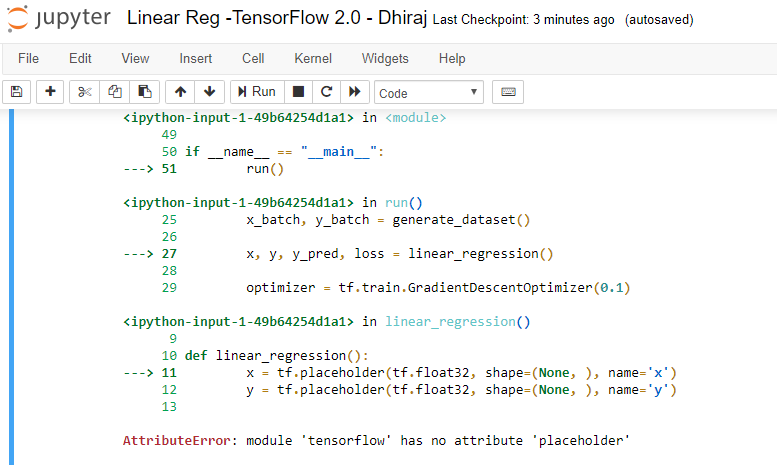If you want to run the existing code (written in version 1.x) with version 2.0, you have two options:

1. Run your TensorFlow 2.0 installation in version 1.0 mode by using the below two lines of code:
``````import tensorflow.compat.v1 as tf
tf.disable_v2_behavior()
``````
1. Modify your code to to work with version 2.0, as well as use the new and exciting features of version 2.0.

## Linear Regression with TensorFlow 2.0

In this article, we’re going to use TensorFlow 2.0-compatible code to train a linear regression model.

Linear regression is an algorithm that finds a linear relationship between a dependent variable and one or more independent variables. The dependent variable is also called a label and independent variables are called features.

We’ll start by importing the necessary libraries. Let’s import three, namely `numpy`, `tensorflow`, and `matplotlib`, as shown below:

``````import numpy as np
import tensorflow as tf
import matplotlib.pyplot as plt
``````

Before coding further, let’s make sure we’ve got the current version of TensorFlow ready to go.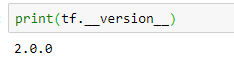Our next step is to create synthetic data for the model, as shown below. Assuming the equation of a line as y = mx + c , note that we’ve taken the slope of the line m as 2 and constant value c as 0.9. There is some error data we’ve introduced using `np.random`, as we don’t want the model to overfit as a straight line — this is because we want the model to work on unseen data.

``````# actual weight = 2 and actual bias = 0.9
x = np.linspace(0, 3, 120)
y = 2 * x + 0.9 + np.random.randn(*x.shape) * 0.3
``````

Let’s plot the data to see if it has linear pattern. We’re using Matplotlib for plotting. The data points below clearly show a pattern we’re looking for. Noticed that the data isn’t a perfectly straight line.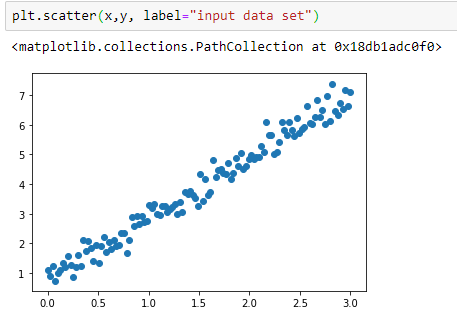After visualizing our data, let’s create a class called `Linear Model` that has two methods: init and call. Init initializes the weight and bias randomly, and call returns the values, as per the straight line equation y = mx + c

``````class LinearModel:
def __call__(self, x):
return self.Weight * x + self.Bias

def __init__(self):
self.Weight = tf.Variable(11.0)
self.Bias = tf.Variable(12.0)
``````

Now let’s define the loss and train functions for the model. The train function takes four parameters: `linear_model` (model instance) , `x` (independent variable) , `y` (dependent variable), and `lr` (learning rate).

The loss function takes two parameters: `y` (actual value of dependent variable) and `pred` (predicted value of dependent variable).

Note that we’re using the `tf.square` function to get the square of the difference of `y` and the predicted value, and then we’re using the . `tf.reduce_mean` method to calculate the square root of the mean.

Note that the `tf.GradientTape` method is used for automatic differentiation, computing the gradient of a computation with respect to its input variables.
Hence, all operations executed inside the context of a `tf.GradientTape` are recorded.

``````def loss(y, pred):
return tf.reduce_mean(tf.square(y - pred))

def train(linear_model, x, y, lr=0.12):
current_loss = loss(y, linear_model(x))

lr_weight, lr_bias = t.gradient(current_loss, [linear_model.Weight, linear_model.Bias])
linear_model.Weight.assign_sub(lr * lr_weight)
linear_model.Bias.assign_sub(lr * lr_bias)
``````

Here we’re defining the number of epochs as 80 and using a for loop to train the model. Note that we’re printing the epoch count and loss for each epoch using that same for loop. We’ve used 0.12 for learning rate, and we’re calculating the loss in each epoch by calling our loss function inside the for loop as shown below.

``````linear_model = LinearModel()
Weights, Biases = [], []
epochs = 80
for epoch_count in range(epochs):
Weights.append(linear_model.Weight.numpy())
Biases.append(linear_model.Bias.numpy())
real_loss = loss(y, linear_model(x))
train(linear_model, x, y, lr=0.12)
print(f"Epoch count {epoch_count}: Loss value: {real_loss.numpy()}")
``````

Below is the output during model training. This shows how our loss value is decreasing as the epoch count is increasing. Note that, initially, the loss was very high as we initialized the model with random values for weight and bias. Once the model starts learning, the loss starts decreasing.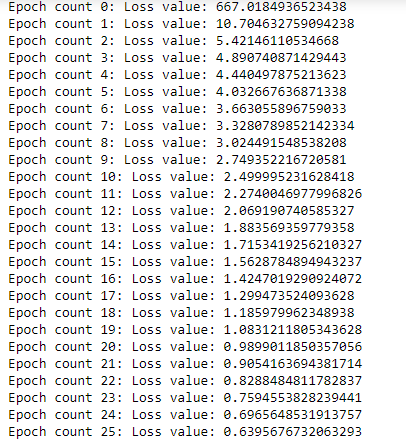And finally, we’d like to know the weight and bias values as well as RMSE for the model, which is shown below.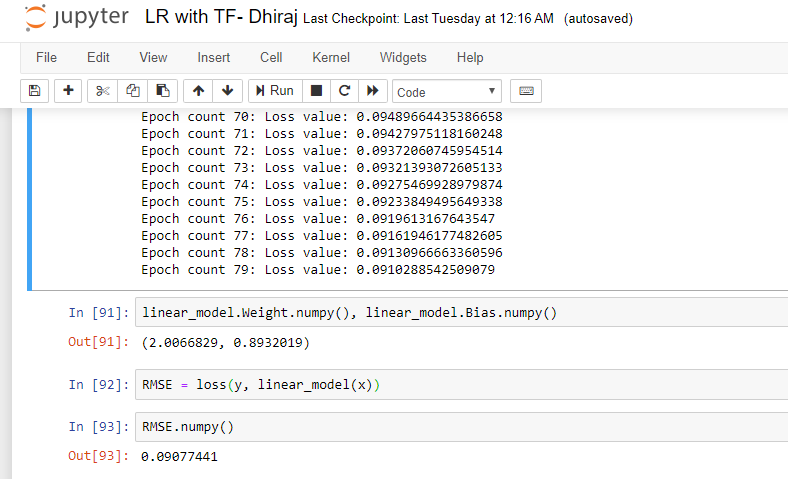## End notes

I hope you enjoyed creating and evaluating a linear regression model with TensorFlow 2.0.

You can find the complete code here.

Happy Machine Learning :)

#tensorflow #machine-learning #python

## Buddha Community1631544191

## Let Developers Just Need to Grasp only One Button Component

From then on, developers only need to master one Button component, which is enough.

Author：Newton(coorchice.cb@alibaba-inc.com)

✨ Features

Rich corner effect

Exquisite border decoration

Flexible icon support

Cool interaction Special effects

High-quality Neumorphism style

🛠 Guide

## 📺 Demo

### 🔩 Basic Demo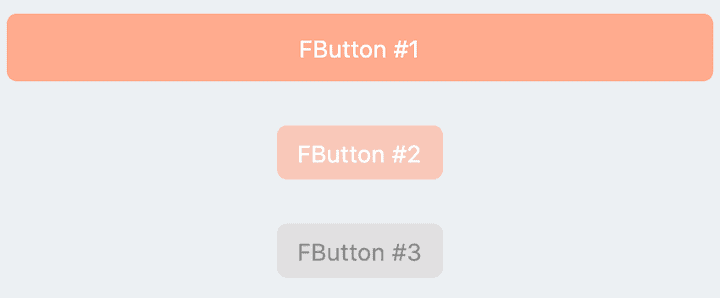``````// FButton #1
FButton(
height: 40,
alignment: Alignment.center,
text: "FButton #1",
style: TextStyle(color: Colors.white),
color: Color(0xffffab91),
onPressed: () {},
)

// FButton #2
FButton(
padding: const EdgeInsets.fromLTRB(12, 8, 12, 8),
text: "FButton #2",
style: TextStyle(color: Colors.white),
color: Color(0xffffab91),
corner: FCorner.all(6.0),
)

// FButton #3
FButton(
padding: const EdgeInsets.fromLTRB(12, 8, 12, 8),
text: "FButton #3",
style: TextStyle(color: Colors.white),
disableStyle: TextStyle(color: Colors.black38),

/// set disable Color
disabledColor: Colors.grey,
corner: FCorner.all(6.0),
)
``````

By simply configuring `text` and`onPressed`, you can construct an available FButton.

If `onPressed` is not set, FButton will be automatically recognized as not unavailable. At this time, ** FButton ** will have a default unavailable status style.

You can also freely configure the style of FButton when it is not available via the `disabledXXX` attribute.

### 🎈 Corner & Stroke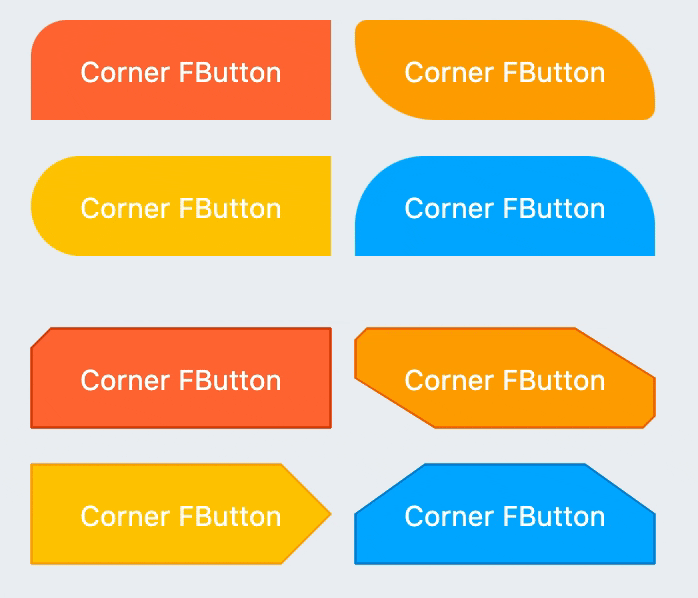``````// #1
FButton(
width: 130,
text: "FButton #1",
style: TextStyle(color: Colors.white),
color: Color(0xffFF7043),
onPressed: () {},
clickEffect: true,

/// 配置边角大小
///
/// set corner size
corner: FCorner.all(25),
),

// #2
FButton(
width: 130,
text: "FButton #2",
style: TextStyle(color: Colors.white),
color: Color(0xffFFA726),
onPressed: () {},
clickEffect: true,
corner: FCorner(
leftBottomCorner: 40,
leftTopCorner: 6,
rightTopCorner: 40,
rightBottomCorner: 6,
),
),

// #3
FButton(
width: 130,
text: "FButton #3",
style: TextStyle(color: Colors.white),
color: Color(0xffFFc900),
onPressed: () {},
clickEffect: true,
corner: FCorner(leftTopCorner: 10),

/// 设置边角风格
///
/// set corner style
cornerStyle: FCornerStyle.bevel,
strokeWidth: 0.5,
strokeColor: Color(0xffF9A825),
),

// #4
FButton(
width: 130,
text: "FButton #4",
style: TextStyle(color: Colors.white),
color: Color(0xff00B0FF),
onPressed: () {},
clickEffect: true,
corner: FCorner(
rightTopCorner: 25,
rightBottomCorner: 25),
cornerStyle: FCornerStyle.bevel,
strokeWidth: 0.5,
strokeColor: Color(0xff000000),
),
``````

You can add rounded corners to FButton via the `corner` property. You can even control each fillet individually。

By default, the corners of FButton are rounded. By setting `cornerStyle: FCornerStyle.bevel`, you can get a bevel effect.

FButton supports control borders, provided that `strokeWidth> 0` can get the effect 🥳.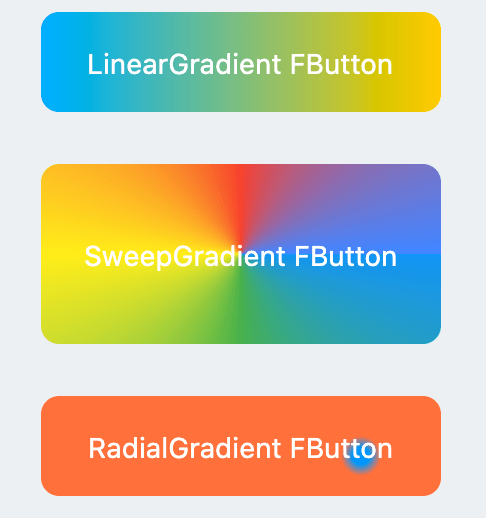``````
FButton(
width: 100,
height: 60,
text: "#1",
style: TextStyle(color: Colors.white),
color: Color(0xffFFc900),

/// 配置渐变色
///
Color(0xff00B0FF),
Color(0xffFFc900),
]),
onPressed: () {},
clickEffect: true,
corner: FCorner.all(8),
)
``````

Through the `gradient` attribute, you can build FButton with gradient colors. You can freely build many types of gradient colors.

### 🍭 Icon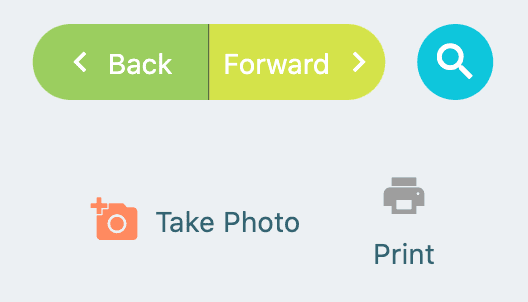``````FButton(
width: 88,
height: 38,
text: "Back",
style: TextStyle(color: Colors.white),
color: Color(0xffffc900),
onPressed: () {
toast(context, "Back!");
},
clickEffect: true,
corner: FCorner(
leftTopCorner: 25,
leftBottomCorner: 25,),

/// 配置图标
///
/// set icon
image: Icon(
Icons.arrow_back_ios,
color: Colors.white,
size: 12,
),

/// 配置图标与文字的间距
///
/// Configure the spacing between icon and text
imageMargin: 8,
),

FButton(
onPressed: () {},
image: Icon(
Icons.print,
color: Colors.grey,
),
imageMargin: 8,

/// 配置图标与文字相对位置
///
/// Configure the relative position of icons and text
imageAlignment: ImageAlignment.top,
text: "Print",
style: TextStyle(color: textColor),
color: Colors.transparent,
),
``````

The `image` property can set an image for FButton and you can adjust the position of the image relative to the text, through`imageAlignment`.

If the button does not need a background, just set `color: Colors.transparent`.

### 🔥 Effect``````
FButton(
width: 200,
text: "Try Me!",
style: TextStyle(color: textColor),
color: Color(0xffffc900),
onPressed: () {},
clickEffect: true,
corner: FCorner.all(9),

/// 配置按下时颜色
///
/// set pressed color
highlightColor: Color(0xffE65100).withOpacity(0.20),

/// 配置 hover 状态时颜色
///
/// set hover color
hoverColor: Colors.redAccent.withOpacity(0.16),
),
``````

The highlight color of FButton can be configured through the `highlightColor` property。

`hoverColor` can configure the color when the mouse moves to the range of FButton, which will be used during Web development.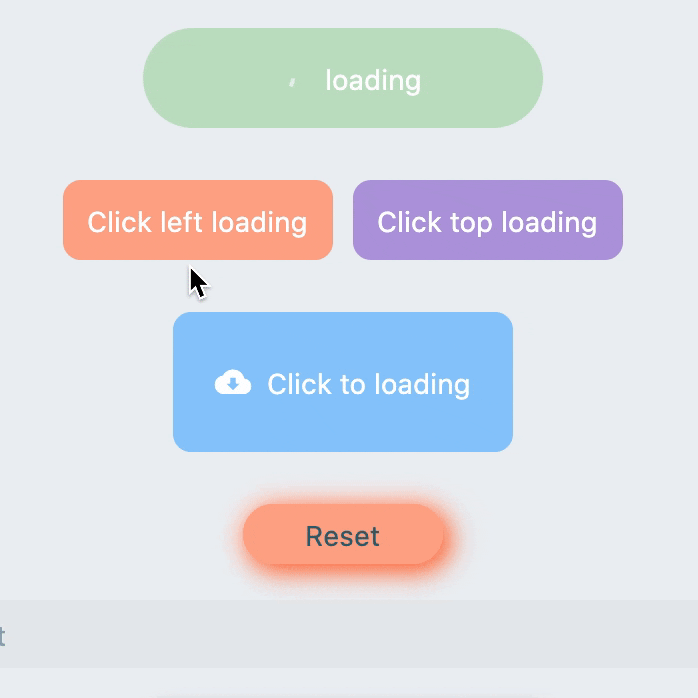``````FButton(
style: TextStyle(color: textColor),
color: Color(0xffffc900),
...

///

///
imageMargin: 6,

///

///

///

///

///
imageAlignment: ImageAlignment.top,
),

// #2
FButton(
width: 170,
height: 70,
style: TextStyle(color: textColor),
color: Color(0xffffc900),
onPressed: () { },
...
imageMargin: 8,

///
)

FButton(
width: 170,
height: 70,
alignment: Alignment.center,
style: TextStyle(color: Colors.white),
color: Color(0xff90caf9),
...
imageMargin: 8,

///
),
``````

Through the `loading` attribute, you can configure Loading effects for ** FButton **.

When FButton is in Loading state, FButton will enter an unavailable state, onPress will no longer be triggered, and unavailable styles will also be applied.

At the same time `loadingText` will overwrite`text` if it is not null.

The click start Loading effect can be achieved through the `clickLoading` attribute.

The position of `loading` will be affected by the`imageAlignment` attribute.

When `hideTextOnLoading: true`, if FButton is in`loading` state, its text will be hidden.

Through `loadingWidget`, developers can set completely customized loading styles.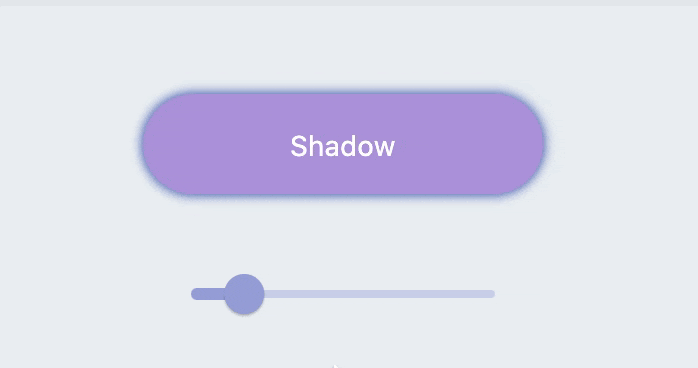``````
FButton(
width: 200,
textColor: Colors.white,
color: Color(0xffffc900),
onPressed: () {},
clickEffect: true,
corner: FCorner.all(28),

/// 配置阴影颜色
///

/// 设置组件高斯与阴影形状卷积的标准偏差。
///
/// Sets the standard deviation of the component's Gaussian convolution with the shadow shape.
),
``````

FButton allows you to configure the color, size, and position of the shadow.

### 🍭 Neumorphism Style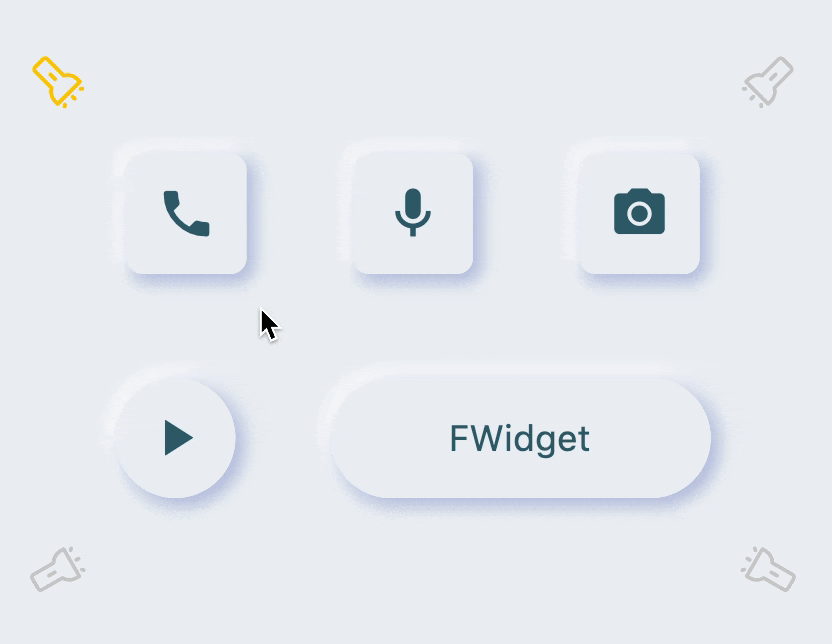``````FButton(

/// 开启 Neumorphism 支持
///
/// Turn on Neumorphism support
isSupportNeumorphism: true,

/// 配置光源方向
///
/// Configure light source direction
lightOrientation: lightOrientation,

/// 配置亮部阴影
///

/// 配置暗部阴影
///
strokeColor: mainBackgroundColor,
strokeWidth: 3.0,
width: 190,
height: 60,
text: "FWidget",
style: TextStyle(
color: mainTextTitleColor, fontSize: neumorphismSize_2_2),
alignment: Alignment.center,
color: mainBackgroundColor,
...
)
``````

FButton brings an incredible, ultra-high texture Neumorphism style to developers.

Developers only need to configure the `isSupportNeumorphism` parameter to enable and disable the Neumorphism style.

If you want to adjust the style of Neumorphism, you can make subtle adjustments through several attributes related to Shadow, among which:

FButton also provides `lightOrientation` parameters, and even allows developers to adjust the care angle, and has obtained different Neumorphism effects.

😃 How to use？

Add dependencies in the project `pubspec.yaml` file:

## 🌐 pub dependency

``````dependencies:
fbutton: ^<version number>
``````

## 🖥 git dependencies

``````dependencies:
fbutton:
git:
url: 'git@github.com:Fliggy-Mobile/fbutton.git'
ref: '<Branch number or tag number>'
``````

## Use this package as a library

### Depend on it

Run this command:

With Flutter:

`` \$ flutter pub add fbutton_nullsafety``

This will add a line like this to your package's pubspec.yaml (and run an implicit `flutter pub get`):

``````dependencies:
fbutton_nullsafety: ^5.0.0``````

Alternatively, your editor might support or `flutter pub get`. Check the docs for your editor to learn more.

### Import it

Now in your Dart code, you can use:

``import 'package:fbutton_nullsafety/fbutton_nullsafety.dart';``

Author: Fliggy-Mobile

Source Code: https://github.com/Fliggy-Mobile/fbutton1594271340

## A Deep Dive into Linear Regression

Let’s begin our journey with the truth — machines never learn. What a typical machine learning algorithm does is find a mathematical equation that, when applied to a given set of training data, produces a prediction that is very close to the actual output.

Why is this not learning? Because if you change the training data or environment even slightly, the algorithm will go haywire! Not how learning works in humans. If you learned to play a video game by looking straight at the screen, you would still be a good player if the screen is slightly tilted by someone, which would not be the case in ML algorithms.

However, most of the algorithms are so complex and intimidating that it gives our mere human intelligence the feel of actual learning, effectively hiding the underlying math within. There goes a dictum that if you can implement the algorithm, you know the algorithm. This saying is lost in the dense jungle of libraries and inbuilt modules which programming languages provide, reducing us to regular programmers calling an API and strengthening further this notion of a black box. Our quest will be to unravel the mysteries of this so-called ‘black box’ which magically produces accurate predictions, detects objects, diagnoses diseases and claims to surpass human intelligence one day.

We will start with one of the not-so-complex and easy to visualize algorithm in the ML paradigm — Linear Regression. The article is divided into the following sections:

1. Need for Linear Regression

2. Visualizing Linear Regression

3. Deriving the formula for weight matrix W

4. Using the formula and performing linear regression on a real world data set

Note: Knowledge on Linear Algebra, a little bit of Calculus and Matrices are a prerequisite to understanding this article

Also, a basic understanding of python, NumPy, and Matplotlib are a must.

## 1) Need for Linear regression

Regression means predicting a real valued number from a given set of input variables. Eg. Predicting temperature based on month of the year, humidity, altitude above sea level, etc. Linear Regression would therefore mean predicting a real valued number that follows a linear trend. Linear regression is the first line of attack to discover correlations in our data.

Now, the first thing that comes to our mind when we hear the word linear is, a line.

Yes! In linear regression, we try to fit a line that best generalizes all the data points in the data set. By generalizing, we mean we try to fit a line that passes very close to all the data points.

But how do we ensure that this happens? To understand this, let’s visualize a 1-D Linear Regression. This is also called as Simple Linear Regression

#calculus #machine-learning #linear-regression-math #linear-regression #linear-regression-python #python1624418820

## Spring Integration AWS 2.5.1 and Spring Cloud Stream Kinesis Binder 2.2.0 Available

Dear Spring Community,

Today it’s my pleasure to announce releases of Spring Integration for Amazon Web Services extension versions `2.5.1` and Spring Cloud Stream Binder for AWS Kinesis `2.2.0`.

``````compile 'org.springframework.integration:spring-integration-aws:2.5.1'
COPY
``````

If you don’t use Kinesis Binder. Or via Binder dependency:

``````compile 'org.springframework.cloud:spring-cloud-stream-binder-kinesis:2.2.0'
COPY
``````

Spring Integration for AWS extension of `2.5.1` has some minor bug fixes after the previous `2.5.0` announcement.

The Spring Cloud Stream Binder for AWS Kinesis `2.2.0` is the first release in the project generation which is based on a new independent Spring Cloud for AWS artifact. It also was upgraded to the latest Spring Cloud 2020.0.3 and has some other bug fixes and improvements.

#[object object] #aws 2.5.1 #spring cloud #spring cloud stream kinesis binder 2.2.0 available #spring integration aws 2.5.1 and spring cloud stream kinesis binder 2.2.0 available1598352300

## Regression: Linear Regression

Machine learning algorithms are not your regular algorithms that we may be used to because they are often described by a combination of some complex statistics and mathematics. Since it is very important to understand the background of any algorithm you want to implement, this could pose a challenge to people with a non-mathematical background as the maths can sap your motivation by slowing you down.In this article, we would be discussing linear and logistic regression and some regression techniques assuming we all have heard or even learnt about the Linear model in Mathematics class at high school. Hopefully, at the end of the article, the concept would be clearer.

**Regression Analysis **is a statistical process for estimating the relationships between the dependent variables (say Y) and one or more independent variables or predictors (X). It explains the changes in the dependent variables with respect to changes in select predictors. Some major uses for regression analysis are in determining the strength of predictors, forecasting an effect, and trend forecasting. It finds the significant relationship between variables and the impact of predictors on dependent variables. In regression, we fit a curve/line (regression/best fit line) to the data points, such that the differences between the distances of data points from the curve/line are minimized.

#regression #machine-learning #beginner #logistic-regression #linear-regression #deep learning1592023980

## 5 Regression algorithms: Explanation & Implementation in Python

Take your current understanding and skills on machine learning algorithms to the next level with this article. What is regression analysis in simple words? How is it applied in practice for real-world problems? And what is the possible snippet of codes in Python you can use for implementation regression algorithms for various objectives? Let’s forget about boring learning stuff and talk about science and the way it works.

#linear-regression-python #linear-regression #multivariate-regression #regression #python-programming